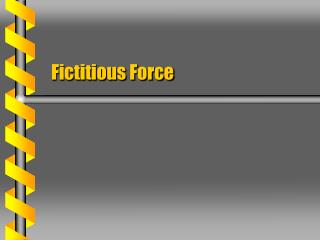DownloadDownload PresentationFictitious Force

# Fictitious Force

Download Presentation## Fictitious Force

- - - - - - - - - - - - - - - - - - - - - - - - - - - E N D - - - - - - - - - - - - - - - - - - - - - - - - - - -
##### Presentation Transcript

1. Fictitious Force

2. The Lagrangian is defined in an inertial system. Follows Newton’s laws Equivalent in other inertial systems Hamilton’s equations are similarly unaffected by a change to another inertial system. Inertial Lagrangian

3. Accelerating Coordinates • Select Cartesian coordinates with acceleration. • Transform along that coordinate • The kinetic energy will be changed. a x2 x2’ x1’ x1

4. Let the accelerated system have no external force. Zero potential The Euler-Lagrange equations have a force-like term. Apparent potential This is a fictitious force. Only the accelerated frame No External Force

5. Rotating Coordinates • A rotating coordinate system is non-inertial. • Time-dependent angle • Transform coordinates • Transform velocities x2’ x2 x1’ wt x1

6. Rewrite the kinetic energy in terms of the rotating coordinates. Summation rule used Find the three EL equations. Rotating Motion

7. The extra terms can be expressed as a vector product. Define the angular velocity w along 3-axis. Product terms only for 1- and 2-axes Wedge Products

8. The vector products can be used to create a vector equation. In the rotating frame there are two fictitious forces unrelated to the potential. Centrifugal force Coriolis force Vector Form next